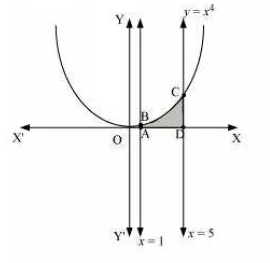# NCERT Solutions for Class 12 Maths Chapter 8 Application of integrals

NCERT Solutions for Class 12 Maths Chapter 8 Application of Integrals: In geometry, you must have learnt some formulas to calculate areas of simple geometrical figures like triangles, rectangles, trapeziums, circles, etc. But how to calculate are areas enclosed by curves? In this article, you will get  NCERT solutions for class 12 maths chapter 8 application of integrals in which such calculations are made easier. Important topics that are going to be discussed in this chapter are the area under simple curves, an area between lines and arcs of circles, parabolas, and ellipses. In CBSE NCERT solutions for class 12 maths chapter 8 applications of integrals article, questions from all these topics are covered. In chapter 7 Integrals, you have learnt integration by finding the area of the bounded curve in the definite integral. Integration has a lot of applications in the field of physics, chemistry, medical science, and real life.  In solutions of NCERT for class 12 maths application of integrals, you will learn some important applications in mathematics. Check all NCERT solutions from class 6 to 12 at a single place, which will help you to learn CBSE maths and science.

Generally, one question( 5 marks) is asked from this chapter in the 12th board final exam and you can score these 5 marks very easily with help of these CBSE NCERT solutions for class 12 maths chapter 8 application of integrals. In this chapter, there are 2 exercises with 20 questions. In the solutions of NCERT for class 12 maths chapter 8 application of integrals, these questions are prepared and explained in a detailed manner using diagrams. Before going to these solutions, first you should try to solve every NCERT problems including examples by yourself. There are total of 14 solved examples are given in the NCERT textbook to give a better understanding of the concepts. At the end of the chapter, 19 questions are given in a miscellaneous exercise. In the CBSE NCERT solutions for class 12 maths chapter 8 application of integrals article, you will get solutions to miscellaneous exercise too.

Let's understand these topics with help of examples

• How to find the area under simple curves-To find the area bounded by the curve   and the ordinates and . Let assume that area under the curve as composed of large numbers of very thin vertical strips. Consider an arbitrary strip of width dx and height y ,then area of the elementary strip(dA) = ydx, where, y = f(x). This small area called the elementary area.• How to find the area of the region bounded by a curve and a line- In this subsection, we will find the area of the region bounded by a line and a circle, a line and an ellipse, a line and a parabola. Equations of above-said curves will be in their standard forms only.

## Topics and sub-topics of NCERT Grade 12 Maths Chapter-8 Application of integrals-

8.1 Introduction

8.2 Area under Simple Curves

8.2.1 The area of the region bounded by a curve and a line

8.3 Area between Two Curves

## CBSE NCERT solutions for class 12 math chapter 8 applications of integrals Exercise: 8.1

Area of the region bounded by the curve and the lines   and the  -axis in the first quadrant

Area  =

= 14/3 units

Area of the region bounded by the curve and the  -axis in the first quadrant

Area  =

units

The area bounded by the curves    and the -axis in the first  quadrant is ABCD.

The area bounded by the ellipse :Area will be 4 times the area of EAB.

Therefore,

Therefore the area bounded by the ellipse will be

The area bounded by the ellipse :The area will be 4 times the area of EAB.

Therefore,

Therefore the area bounded by the ellipse will be

The area of the region bounded by  and   is ABC shown:The point B of the intersection of the line and the circle in the first quadrant is .

Area ABC = Area ABM + Area BMC where, M is point in x-axis perpendicular drawn from the line.

Now,area of      ............(1)

and Area of

..................................(2)

then adding the area (1) and (2), we have then

The Area under ABCwe need to find the area of smaller part of the circle
Now,
Area of ABCD = 2 X Area of ABC
Area of ABC =

Area of ABCD = 2 X Area of ABC

Therefore, the area of the smaller part of the circle iswe can clearly see that given area is symmetrical about x - axis
It is given that
Area of OED = Area of EFCD
Area of OED =
Area of EFCD =
Area of OED = Area of EFCD

Therefore, the value of a isWe can clearly see that given area is symmetrical about y-axis
Therefore,
Area of OCAO = Area of OBDO
Point of intersection of   is  (1 , 1)  and (-1 , 1)
Now,
Area od OCAO = Area OAM - Area of OCMO
Area of OAM =
Area of OCMO =
Therefore,
Area od OCAO
Now,
Area of the region bounded by the parabola  and  is = 2 X Area od OCAO    UnitsPoints of intersections of  is

Now,
Area of OBAO = Area of OBCO + Area of OCAO
Area of OBCO = Area of OMBCO- Area of OMBO

Area of OMBCO =

Area of OMBO =

Area of OBCO = Area of OMBCO- Area of OMBO

Similarly,
Area  of OCAO =  Area of  OCALO - Area of OALO

Area of  OCALO =

Area of OALO =

Area  of OCAO =  Area of  OCALO - Area of OALO

Now,
Area of OBAO = Area of OBCO + Area of OCAO

Therefore, area bounded by the curve  and the line   is

The combined figure of the curve  and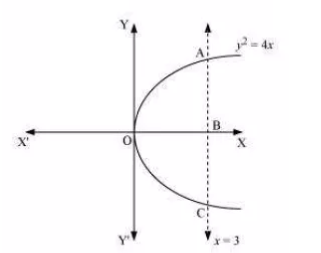The required are is OABCO, and it is symmetrical about the horizontal axis.
Therefore, Area of OABCO = 2 Area of OAB

therefore the required area is  units.

Area lying in the first quadrant and bounded by the circle   and the lines and     is

The area bounded by circle C(0,0,4) and the line x=2 is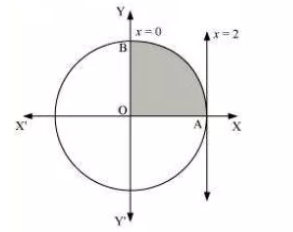The required area = area of OAB

Question: 13 Choose the correct answer in the following.

Area of the region bounded by the curve , -axis and the line   is

(A)                 (B)                  (C)                 (D)

The area bounded by the curve  and y =3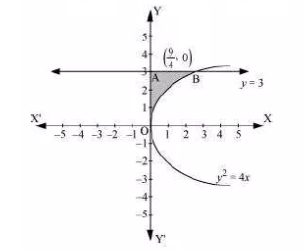the required area = OAB =

## Solutions of NCERT for class 12 maths chapter 8 application of integrals Exercise: 8.2

The area bounded by the circle  and the parabola  .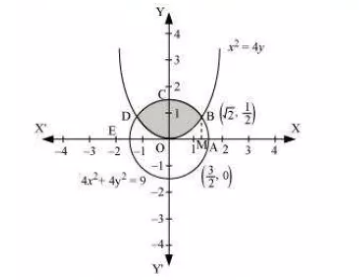By solving the equation we get the intersecting point  and
So, the required area (OBCDO)=2 times the area of (OBCO)
Draw a normal on the x-axis (M =)

Thus the area of OBCO = Area of OMBCO - Area of OMBO

S0, total area =Given curves are     and

Point of intersection of these two curves are

and

We can clearly see that the required area is symmetrical about the x-axis

Therefore,

Area of OBCAO = 2   Area of OCAO

Now, join AB such that it intersects the x-axis at M and AM is perpendicular to OC

Coordinates of M =

Now,

Area OCAO = Area OMAO + Area CMAC

Now,
Area of OBCAO = 2   Area of OCAO

The area of the region bounded by the curves,

and  is represented by the shaded area OCBAO asThen, Area OCBAO will be = Area of ODBAO - Area of ODCO

which is equal to

So, we draw BL and CM perpendicular to x-axis.

Then it can be observed in the following figure that,

We have the graph as follows:Equation of the line segment AB is:

or

Therefore we have Area of

So, the equation of line segment BC is

or

Therefore the area of BLMCB will be,

Equation of the line segment AC is,

or

Therefore the area of AMCA will be,

Therefore, from equations (1), we get

The area of the triangle

The equations of sides of the triangle are .

ON solving these equations, we will get the vertices of the triangle asThus it can be seen that,

Smaller area enclosed by the circle   and the line    is

(A)          (B)          (C)         (D)

So, the smaller area enclosed by the circle, , and the line, , is represented by the shaded area ACBA asThus it can be observed that,

Area of ACBA = Area OACBO - Area of

Thus, the correct answer is B.

Area lying between the curves and is

(A)               (B)              (C)              (D)

The area lying between the curve,  and is represented by the shaded area OBAO asThe points of intersection of these curves are  and .

So, we draw AC perpendicular to x-axis such that the coordinates of C are (1,0).

Therefore the Area OBAO =

Thus the correct answer is B.

## NCERT solutions for class 12 maths chapter 8 application of integrals Miscellaneous: Exercise

(i)   and -axis

The area bounded by the curve   and -axis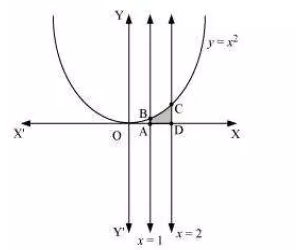The area of the required region = area of ABCD

Hence the area of shaded region is 7/3 units

(ii)   and -axis